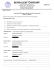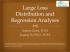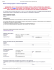# Missing Data Techniques with SAS

## Transcription

Missing Data Techniques with SAS
```MISSING DATA
TECHNIQUES
WITH SAS
IDRE
Statistical
Consulting
Group
To discuss:
1. Commonly used techniques for handling missing
data, focusing on multiple imputation
2. Issues that could arise when these techniques are
used
3. Implementation of SAS Proc MI procedure
 Assuming MVN
 Assuming FCS
4. Imputation Diagnostics
GOALS OF STATISTICAL ANALYSIS WITH
MISSING DATA
Minimize bias
Maximize use of available
information
Obtain appropriate estimates of
uncertainty
THE MISSING DATA MECHANISM DESCRIBES THE
PROCESS THAT IS BELIEVED TO HAVE GENERATED
THE MISSING VALUES.
1.
Missing completely at random (MCAR)
 Neither the unobserved values of the variable with missing nor
the other variables in the dataset predict whether a value will be
missing.
 Example: Planned missingness
2.
Missing at random (MAR)
 Other variables (but not the variable with missing itself) in the
dataset can be used to predict missingness.
 Example: Men may be more likely to decline to answer some
questions than women
3.
Missing not at random (MNAR)
 The unobserved value of the variable with missing predicts
missingness.
 Example: Individuals with very high incomes are more likely to
OUR DATA
High School and Beyond
N=200
13 Variables
Student Demographics and
Achievement including test
scores
ANALYSIS OF FULL DATA
COMMON TECHNIQUES FOR DEALING
WITH MISSING DATA
1.
2.
3.
4.
Complete case analysis (listwise deletion)
Mean Imputation
Single Imputation
Stochastic Imputation
COMPLETE CASE ANALYSIS
(LISTWISE DELETION)
Method: Drop cases with missing data on any
variable of interest
Appeal: Nothing to implement – default
method
Drawbacks:
 Loss of cases/data
 Biased estimates unless MCAR
COMPLETE CASE ANALYSIS
(LISTWISE DELETION)
 proc means data = ats.hsb_mar nmiss N min max mean std;
var _numeric_ ;
run;
LISTWISE DELETION ANALYSIS DROPS
OBSERVATIONS WITH MISSING VALUES
UNCONDITIONAL MEAN IMPUTATION
Method: Replace missing values for a variable
with its overall estimated mean
Appeal: Simple and easily implemented
Drawbacks:
 Artificial reduction in variability b/c imputing values
at the mean.
 Changes the magnitude of correlations between the
imputed variables and other variables.
M E A N , SD A N D C OR R E LATI ON M AT R IX OF 5 VA R I ABLES
BE FORE & A F T ER M E A N I M P UTATION
SINGLE OR DETERMINISTIC
(REGRESSION) IMPUTATION
Method: Replace missing values with
predicted scores from a regression equation.
Appeal: Uses complete information to impute
values.
Drawback: All predicted values fall directly on
the regression line, decreasing variability.
Also known as regression imputation
SINGLE OR DETERMINISTIC
(REGRESSION) IMPUTATION
p.46, Applied Missing Data Analysis, Craig Enders (2010)
SINGLE OR DETERMINISTIC
(REGRESSION) IMPUTATION
Imputing values directly on the regression
line:
 Underestimates uncertainty
 Inflates associations between variables because it
imputes perfectly correlated values
 Upwardly biases R-squared statistics, even under the
assumption of MCAR
STOCHASTIC IMPUTATION
 Stochastic imputation addresses these problems
with regression imputation by incorporating or
 Method: Add randomly drawn residual to imputed
value from regression imputation. Distribution of
residuals based on residual variance from regression
model.
STOCHASTIC IMPUTATION
p.48, Applied Missing Data Analysis, Craig Enders (2010)
STOCHASTIC IMPUTATION
Appeals:
Restores some lost variability.
Superior to the previous methods as it will
produce unbiased coefficient estimates under
MAR.
Drawback: SE’s produced during stochastic
estimation, while less biased, will still be
attenuated.
WHAT IS MULTIPLE IMPUTATION?
 Iterative form of stochastic imputation.
 Multiple values are imputed rather than a single value to
reflect the uncertainty around the “true” value.
 Each imputed value includes a random component whose
magnitude reflects the extent to which other variables in
the model cannot predict it's “true “value
 Common misconception: imputed values should
represent "real" values.
 Purpose: To correctly reproduce the full data
variance/covariance matrix
ISN'T MULTIPLE IMPUTATION JUST
MAKING UP DATA?
 No.
 This is argument applies to single imputation methods
 MI analysis methods account for the uncertainty/error
associated with the imputed values.
 Estimated parameters never depend on a single value.
THREE PHASES
 1 . Imputation or Fill-in Phase: Missing values are imputed,
forming a complete data set. This process is repeated m
times.
 2. Analysis Phase: Each of the m complete data sets is then
analyzed using a statistical model (e.g linear regression).
 3. Pooling Phase: The parameter estimates (e.g. coef ficients
and standard errors) obtained from each analyzed data set
are then combined for inference.
THE IMPORTANCE OF BEING COMPATIBLE
 The imputation model should be " congenial“ to or consistent
 Includes, at the very least, the same variables as the analytic model.
 Includes any transformations to variables in the analytic model
 E.g. logarithmic and squaring transformations, interaction terms
 All relationships between variables should be represented and
estimated simultaneously.
 Otherwise, you are imputing values assuming they are
uncorrelated with the variables you did not include.
PREPARING FOR MULTIPLE IMPUTATION
1. Examine the number and proportion of missing values
among your variables of interest .
2. Examine Missing Data Patterns among your variables of
interest.
3. If necessary, identify potential auxiliary variables
4. Determine imputation method
EXAMINE MISSING VALUES: PROC
MEANS NMISS OPTION
EXAMINE MISSING VALUES: NOTE VARIABLE(S ) WITH
HIGH PROPORTION OF MISSING -- THEY WILL IMPACT
MODEL CONVERGENCE THE MOST
EXAMINE MISSING DATA PATTERNS:
SYNTAX
proc mi data=hsb_mar nimpute=0 ;
var write read female math prog ;
ods select misspattern;
run;
EXAMINE MISSING DATA PATTERNS
IDENTIFY POTENTIAL AUXILIARY
VARIABLES
 Characteristics:
 Correlated with missing variable (rule of thumb: r> 0.4)
 Predictor of missingness
 Not of analytic interest, so only used in imputation model
 Why? Including auxiliary variables in the
imputation model can:
 Improve the quality of imputed values
 Increase power, especially with high fraction of missing
information (FMI >25%)
 Be especially important when imputing DV
 Increase plausibility of MAR
HOW DO YOU IDENTIFY
AUXILIARY VARIABLES?
A priori knowledge
Previous literature
Identify associations in data
AUXILIARY VARIABLES ARE CORRELATED
WITH MISSING VARIABLE
AUXILIARY VARIABLES ARE PREDICTORS
OF MISSINGNESS
IMPUTATION MODEL
EXAMPLE 1:
MI USING MULTIVARIATE
NORMAL DISTRIBUTION
(MVN)
ASSUMING A JOINT MULTIVARIATE
NORMAL DISTRIBUTION
 Probably the most common parametric approach for multiple
imputation.
 Assumes variables are individually and jointly normally
distributed
 Assuming a MVN distribution is robust to violations of
normality given a large enough N.
 Uses the data augmentation (DA) algorithm to impute.
 Biased estimates may result when N is relatively small and
the fraction of missing information is high.
IMPUTATION PHASE
proc mi data= new nimpute=10 out=mi_mvn seed=54321;
var socst science write read female math progcat1 progcat2 ;
run;
MULTIPLY IMPUTED DATASET
ANALYSIS PHASE:
ESTIMATE MODEL FOR EACH
IMPUTED DATASET
proc glm data = mi_mvn ;
model read = write female math progcat1
progcat2;
by _imputation_;
ods output ParameterEstimates=a_mvn;
run;
quit;
PARAMETER ESTIMATE DATASET
POOLING PHASECOMBINING PARAMETER ESTIMATES
ACROSS DATASETS
proc mianalyze parms=a_mvn;
modeleffects intercept write female math
progcat1 progcat2;
run;
COMPARE MIANALYZE ESTIMATES
TO ANALYSIS WITH FULL DATA
FULL DATA ANALYSIS
MIANALYZE OUPUT
HOW DOES PROC MIANALYZE WORK
 PROC MIANALYZE combines results across
imputations
 Regression coefficients are averaged across
imputations
 Standard errors incorporate uncertainty from 2
sources:
 "within imputation" - variability in estimate expected
with no missing data
 The usual uncertainty regarding a regression coefficient
 "between imputation" - variability due to missing
information.
 The uncertainty surrounding missing values
OUTPUT FROM MIANALYZE
VARIANCE WITHIN
Sampling variability
expected with no
missing data.
Average of
variability of
coefficients within
an imputation
Equal to arithmetic
mean of sampling
variances (SE 2 )
together 10
estimated SE 2 for
write and divide by
10
V w = 0.006014
VARIANCE BETWEEN
 Variability in
estimates across
imputations
 i.e. the variance of the m
coefficients
 Estimates the
(uncertainty) that
results from missing
data.
 Example: take all 10
of the parameter
estimates (β) for write
and calculate the
variance
 V B = 0.000262.
TOTAL VARIANCE
 The total variance is
sum of 3 sources of
variance.
 Within,
 Between
sampling variance.
 What is the sampling
variance?
 Variance Between
divided by number of
imputations
 Represents sampling
error associated with the
overall coefficient
estimates.
 Serves as a correction
factor for using a
specific number of
imputations.
DEGREES OF FREEDOM
 DF for combined results
are determined by the
number of imputations.
 *By default DF = infinity,
typically not a problem
with large N but can be
with smaller samples
 The standard formula to
estimate DF can yield
estimates that are
fractional or that far
exceed the DF for
complete data.
the problem of inflated
DF has been
implemented
 Use the EDF option on the
proc mianalyze line to
indicate to SAS what is
RELATIVE INCREASES IN VARIANCE (RVI)
Proportional
increase in total
sampling variance
due to missing
information
[V B + V B /m]
Vw
For example, the RVI
for write coefficient
is 0.048, meaning
that the sampling
variance is
4.8% larger than it
would have been
with complete data.
FRACTION OF MISSING INFORMATION
(FMI)
 Directly related to
RVI.
 Proportion of total
sampling variance
that is due to missing
data
[V B + V B /m]
VT
 For a given variable,
FMI based on
percentage missing
and correlations with
other imputation
model variables.
 Interpretation similar
to an R 2 .
 Example: FMI=.046 for
write means that 4.6%
of sampling variance is
attributable to missing
data.
RELATIVE EFFICIENCY:
IS 5 IMPUTATIONS ENOUGH?
 Captures how well true population parameters are
estimated
 Related to both the FMI and m
 Low FMI + few m = high efficiency
 As FMI increase so should m:
 Better statistical power and more stable estimate
DIAGN0STICS:
HOW DO I KNOW IF IT WORKED?
Compare means and frequencies of observed
and imputed values.
Use boxplots to compare distributions
Look at “Variance Information” tables from
the proc mianalyze output
Plots - Assess convergence of DA algorithm
TRACE PLOTS:
DID MY IMPUTATION MODEL CONVERGE?
Convergence for each imputed variable can be
assessed using trace plots.
Examine for each imputed variables
Special attention to variables with a high FMI
proc mi data= ats.hsb_mar nimpute=10
out=mi_mvn;
mcmc plots=trace plots=acf ;
var socst write read female math;
run;
EXAMPLE OF A POOR TRACE PLOT
AUTOCORRELATION PLOTS:
DID MY IMPUTATION MODEL CONVERGE?
Assess possible auto correlation of parameter
values between iterations.
Assess the magnitude of the observed
dependency of imputed values across
iterations.
proc mi data= ats.hsb_mar nimpute=10
out=mi_mvn;
mcmc plots=trace plots=acf ;
var socst write read female math;
run;
IMPUTATION MODEL
EXAMPLE 2:
MI USING FULLY
CONDITIONAL
SPECIFICATION (FCS)
WHAT IF I DON’T WANT TO ASSUME A
MULTIVARIATE NORMAL DISTRIBUTION?
 Alternative method for imputation is Fully
Conditional Method (FCS)
 FCS does not assume a joint distribution and
allows the use of different distributions across
variables.
 Each variable with missing is allowed its own
type of regression (linear, logistic, etc) for
imputation
 Example uses:
 Logistic model for binary outcome
 Poisson model for count variable
 Other bounded values
AVAILABLE DISTRIBUTIONS
 FCS methods available:
 Discriminant function or logistic regression for binary/categorical
variables
 Linear regression and predictive mean matching for continuous
variables.
 Properties to Note:
1. Discriminant function only continuous vars as covariates
(default).
2. Logistic regression assumes ordering of class variables if more
then two levels (default).
3. Regression is default imputation method for continuous vars.
4. PMM will provide “plausible” values.
1.
For an observation missing on X, finds cases in data with similar
values on other covariates, then randomly selects an X value from
those cases
IMPUTATION PHASE
proc mi data= ats.hsb_mar nimpute=20
out=mi_fcs ;
class female prog;
fcs plots=trace(mean std);
var socst write read female math science prog;
fcs discrim(female prog /classeffects=include)
nbiter =100 ;
run;
ALTERNATE EXAMPLE
 proc mi data= ats.hsb_mar nimpute=20
out=mi_new1;
class female prog;
var socst write read female math science prog;
fcs logistic (female= socst science) ;
fcs logistic (prog =math socst /link=glogit)
run;
ANALYSIS PHASE:
ESTIMATE GLM MODEL USING EACH
IMPUTED DATASET
proc genmod data=mi_fcs;
class female prog;
by _imputation_;
ods output ParameterEstimates=gm_fcs;
run;
POOLING PHASECOMBINING PARAMETER ESTIMATES
ACROSS DATASETS
PROC MIANALYZE parms(classvar=level)=gm_fcs;
class female prog;
MODELEFFECTS INTERCEPT write female math
prog;
RUN;
TRACE PLOTS:
DID MY IMPUTATION MODEL CONVERGE?
FCS HAS SEVERAL PROPERTIES THAT
MAKE IT AN ATTRACTIVE ALTERNATIVE
1. FCS allows each variable to be imputed using its
own conditional distribution
2. Different imputation models can be specified for
different variables. However, this can also cause
estimation problems.
Beware: Convergence Issues such as complete and
quasi-complete separation (e.g. zero cells) when
imputing categorical variables.
BOTTOM LINE
MI improves over single imputation methods
because:
 Single value never used
 Appropriate estimates of uncertainty
The nature of your data and model will
determine if you choose MVN or FCS
Both are state of the art methods for handling
missing data
 Produce unbiased estimates assuming MAR
```

### ERRORS IN SAMPLE SURVEYS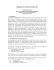### Syllabus for BIOSTAT880 - University of Michigan School of Public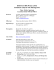### What to Do about Missing Values in Time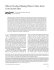### LRTrek - ActFX Algorithmic Trading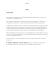### Out of Sight, Not Out of Mind: Strategies for Handling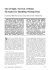### Slides - Center for AIDS Prevention Studies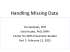### E-communication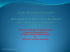### PowerPoint presentation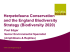### Corsair-V Nicky Camo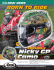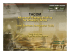### Poster - University of Sussex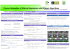### BENSALEM TOWNSHIP